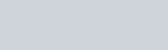6.1.1 for Android
App Name Math Games, Learn Plus, Minus, Multiply & Division v6.1.1 Educational, Games 9.6 MB 6.1.12020-12-03 100,000+

## Math Games, Learn Plus, Minus, Multiply & Division

Game Math Games, Learn Plus, Minus, Multiply & Division nằm trong danh mục Educational

## Giới thiệu Math Games, Learn Plus, Minus, Multiply & Division

The goal behind making this game of Jigar Education Hub team is that you can easily learn mathematics by playing the game. With this educational app parents, teachers and educators can help Student to learn faster.

Math Games
Learn math’s all operation with fun
➖ Subtraction
✖️ Multiplication
➗ Division
√ Square (root )
➕,➖ Mixed operations
👬 Play with friend

Benefits of Using Math Games
• Meets Mathematics Standards
• Easily Linked to Any Mathematics Textbook
• Offers Multiple Assessment Opportunities
• Meets the Needs of Diverse Learners (UA)
• Supports Concept Development in Math
• Encourages Mathematical Reasoning
• Engaging (maintains interest)
• Repeatable (reuse often & sustain involvement
• Open-Ended (allows for multiple approaches & solutions)
• Easy to Prepare
• Easy to Vary for Extended Use & Differentiated Instruction
• Improves Basic Skills
• Enhances Number and Operation Sense
• Encourages Strategic Thinking
• Promotes Mathematical Communication
• Promotes Positive Attitudes Toward Math
• Encourages Parent Involvement

Features
• Addition Games : Simple Addition game to learn math in fun way.
• Subtraction games : Subtracting numbers to solve the equations.
• Multiplication Games : Multiplication Table is useful tool for every one every day.
• Division Games : Enjoy surpassing the division game and learn the divisions table.
Math games for All : Improve your math skills for various mathematical operations like addition, subtraction, multiplication and division.
Brain Training : Here are 60 levels, All levels are suitable for adult and all.
• challenge game : challenge your friend 2 player game.
Cool math games : Cool Math Games provides all kinds of interesting and fun games for players of all ages and skill levels.
• puzzles in maths : Try using math puzzles to get students strategically thinking about math.
• Educational games : Educational games are games that are designed to help people learn about certain subject.
• Math learning : its learning games help you to learn cool math examples.
Multiplication Table : You can learn all the Multiplication tables from 1 to 20 by playing this game.
• Math Practice : By doing many basic examples Practices , you will have much impression of all types examples.
Math Exercises : Learn Add, Subtract, Multiply & Divide

What is a math game ?
• The most effective math games are those in which the structure and rules of the games are based on mathematical ideas, and winning a game is directly related to understanding the mathematics.

• This game is best for him who is looking for cool math games in United States (USA), United Kingdom (UK) and all European countries.

Support 15+ Multiple Languages
• English, Japanese, Chinese simplified, Chinese Traditional, Hindi, Russian, Korean, German, Spanish, Portuguese, Indonesian, French, Italian, Malay, Turkish, Thai.